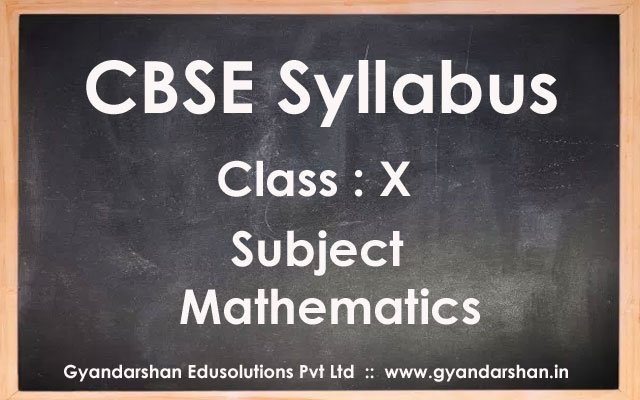Home CBSE Syllabus Mathematics Class X Syllabus

# Mathematics Class X Syllabus

392
0### Exam Structure

 Units Marks I Number Systems 06 II Algebra 20 III Coordinate Geometry 06 IV Geometry 15 V Trigonometry 12 VI Mensuration 10 VII Statistics & Probability 11 Total 80

### UNIT I: NUMBER SYSTEMS

1. REAL NUMBERS

Euclid’s division lemma, Fundamental Theorem of Arithmetic – statements after reviewing work done earlier and after illustrating and motivating through examples, Proofs of results – irrationality of √2, √3, √5, decimal expansions of rational numbers in terms of terminating/non-terminating recurring decimals.

### UNIT II: ALGEBRA

1. POLYNOMIALS

Zeros of a polynomial. Relationship between zeros and coefficients of quadratic polynomials. Statement and simple problems on division algorithm for polynomials with real coefficients.

2. PAIR OF LINEAR EQUATIONS IN TWO VARIABLES

Pair of linear equations in two variables and their graphical solution. Geometric representation of different possibilities of solutions/inconsistency.

Algebraic conditions for number of solutions. Solution of a pair of linear equations in two variables algebraically – by substitution, by elimination and by cross multiplication method. Simple situational problems must be included. Simple problems on equations reducible to linear equations.

Standard form of a quadratic equation ax2+bx+c=0, (a ≠ 0). Solution of the quadratic equations (only real roots) by factorization, by completing the square and by using quadratic formula. Relationship between discriminant and nature of roots.

Situational problems based on quadratic equations related to day to day activities to be incorporated.

4. ARITHMETIC PROGRESSIONS

Motivation for studying Arithmetic Progression Derivation of the nth term and sum of the first n terms of A.P. and their application in solving daily life problems.

### UNIT III: COORDINATE GEOMETRY

1. LINES (In two-dimensions)

Concepts of coordinate geometry, graphs of linear equations. Distance formula. Section formula (internal division). Area of a triangle.

### UNIT IV: GEOMETRY

1. TRIANGLES

Definitions, examples, counter examples of similar triangles.

1. (Prove) If a line is drawn parallel to one side of a triangle to intersect the other two sides in distinct points, the other two sides are divided in the same ratio.
2. (Motivate) If a line divides two sides of a triangle in the same ratio, the line is parallel to the third side.
3. (Motivate) If in two triangles, the corresponding angles are equal, their corresponding sides are proportional and the triangles are similar.
4. (Motivate) If the corresponding sides of two triangles are proportional, their corresponding angles are equal and the two triangles are similar.
5. (Motivate) If one angle of a triangle is equal to one angle of another triangle and the sides including these angles are proportional, the two triangles are similar.
6. (Motivate) If a perpendicular is drawn from the vertex of the right angle of a right triangle to the hypotenuse, the triangles on each side of the perpendicular are similar to the whole triangle and to each other.
7. (Prove) The ratio of the areas of two similar triangles is equal to the ratio of the squares on their corresponding sides.
8. (Prove) In a right triangle, the square on the hypotenuse is equal to the sum of the squares on the other two sides.
9. (Prove) In a triangle, if the square on one side is equal to sum of the squares on the other two sides, the angles opposite to the first side is a right traingle.

2. CIRCLES

Tangents to a circle motivated by chords drawn from points coming closer and closer to the point.

1. (Prove) The tangent at any point of a circle is perpendicular to the radius through the point of contact.
2. (Prove) The lengths of tangents drawn from an external point to circle are equal.

3. CONSTRUCTIONS

1. Division of a line segment in a given ratio (internally).
2. Tangent to a circle from a point outside it.
3. Construction of a triangle similar to a given triangle.

### UNIT V: TRIGONOMETRY

1 . INTRODUCTION TO TRIGONOMETRY

Trigonometric ratios of an acute angle of a right-angled triangle. Proof of their existence (well defined); motivate the ratios, whichever are defined at 0° and 90°. Values (with proofs) of the trigonometric ratios of 30°, 45° and 60°. Relationships between the ratios.

2. TRIGONOMETRIC IDENTITIES

Proof and applications of the identity sin2A + cos2A = 1. Only simple identities to be given. Trigonometric ratios of complementary angles.

3. HEIGHTS AND DISTANCES

Simple and believable problems on heights and distances. Problems should not involve more than two right triangles. Angles of elevation / depression should be only 30°, 45°, 60°.

### UNIT VI: MENSURATION

1. AREAS RELATED TO CIRCLES

Motivate the area of a circle; area of sectors and segments of a circle. Problems based on areas and perimeter / circumference of the above said plane figures. (In calculating area of segment of a circle, problems should be restricted to central angle of 60°, 90° and 120° only. Plane figures involving triangles, simple quadrilaterals and circle should be taken).

2. SURFACE AREAS AND VOLUMES

(i) Problems on finding surface areas and volumes of combinations of any two of the following: cubes, cuboids, spheres, hemispheres and right circular cylinders/cones. Frustum of a cone.

(ii) Problems involving converting one type of metallic solid into another and other mixed problems. (Problems with combination of not more than two different solids be taken).

### UNIT VII: STATISTICS AND PROBABILITY

1. STATISTICS

Mean, median and mode of grouped data (bimodal situation to be avoided). Cumulative frequency graph.

2. PROBABILITY

Classical definition of probability. Simple problems on single events (not using set notation).

Previous articleHindi B Class X Syllabus
Next articleNTSE Class X Syllabus and Exam Pattern